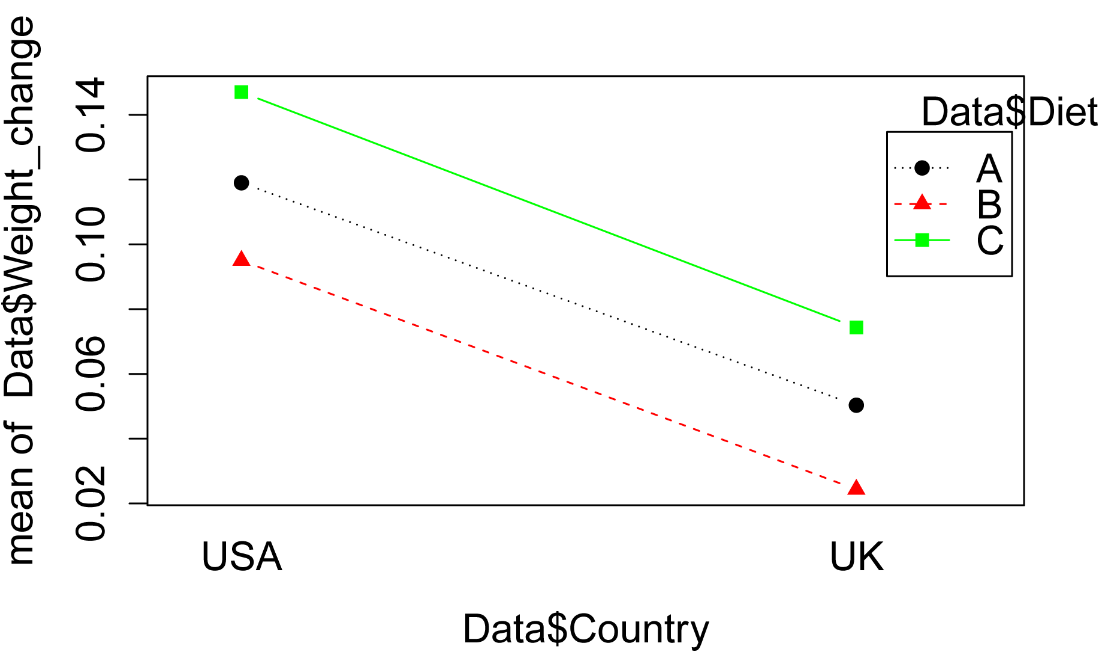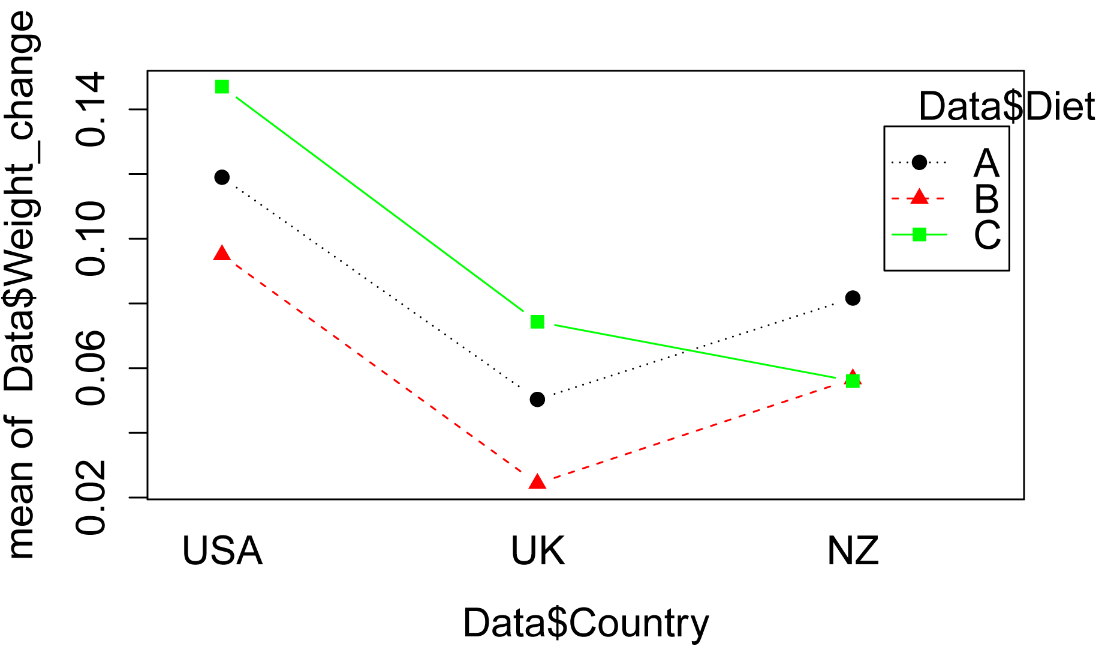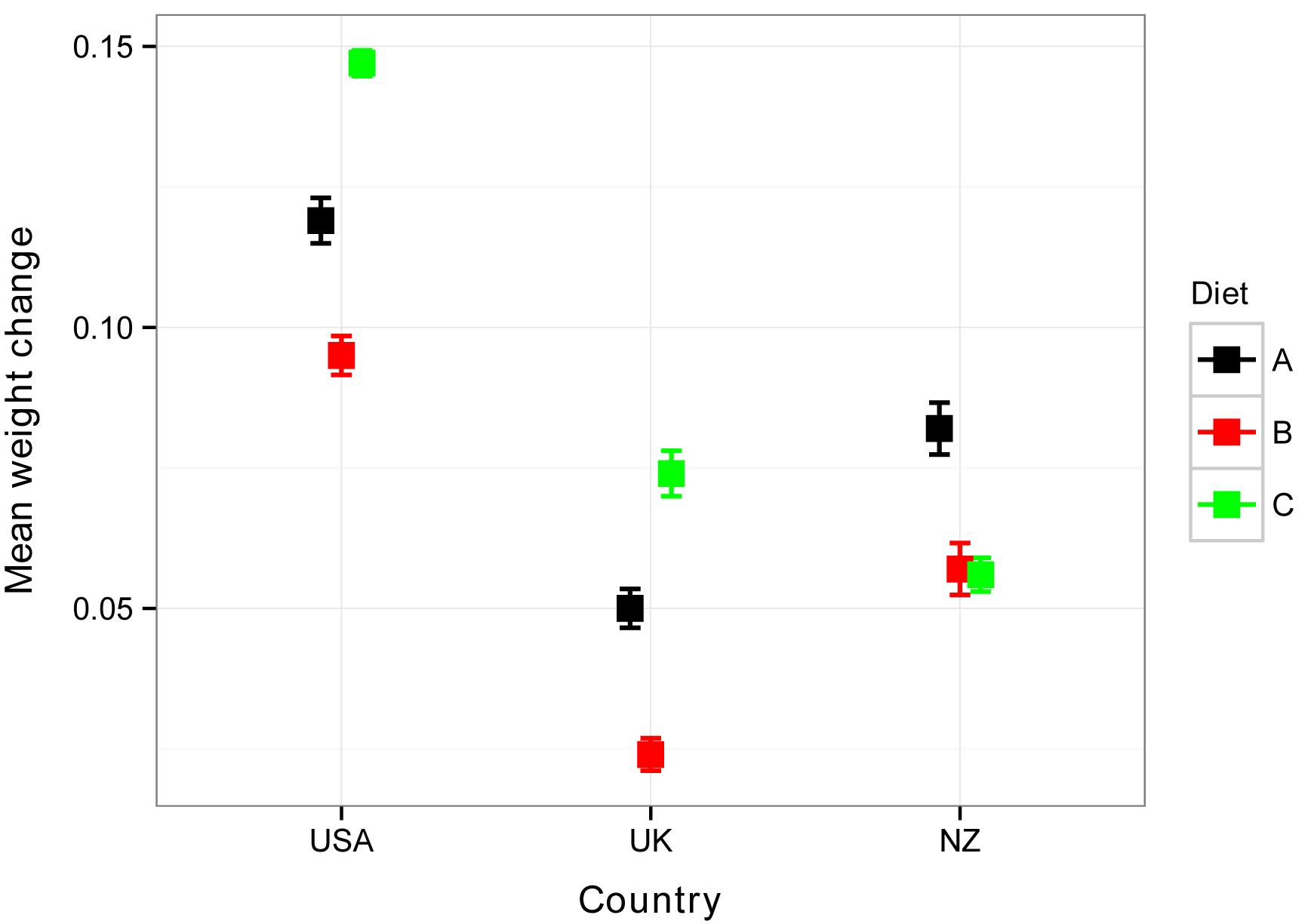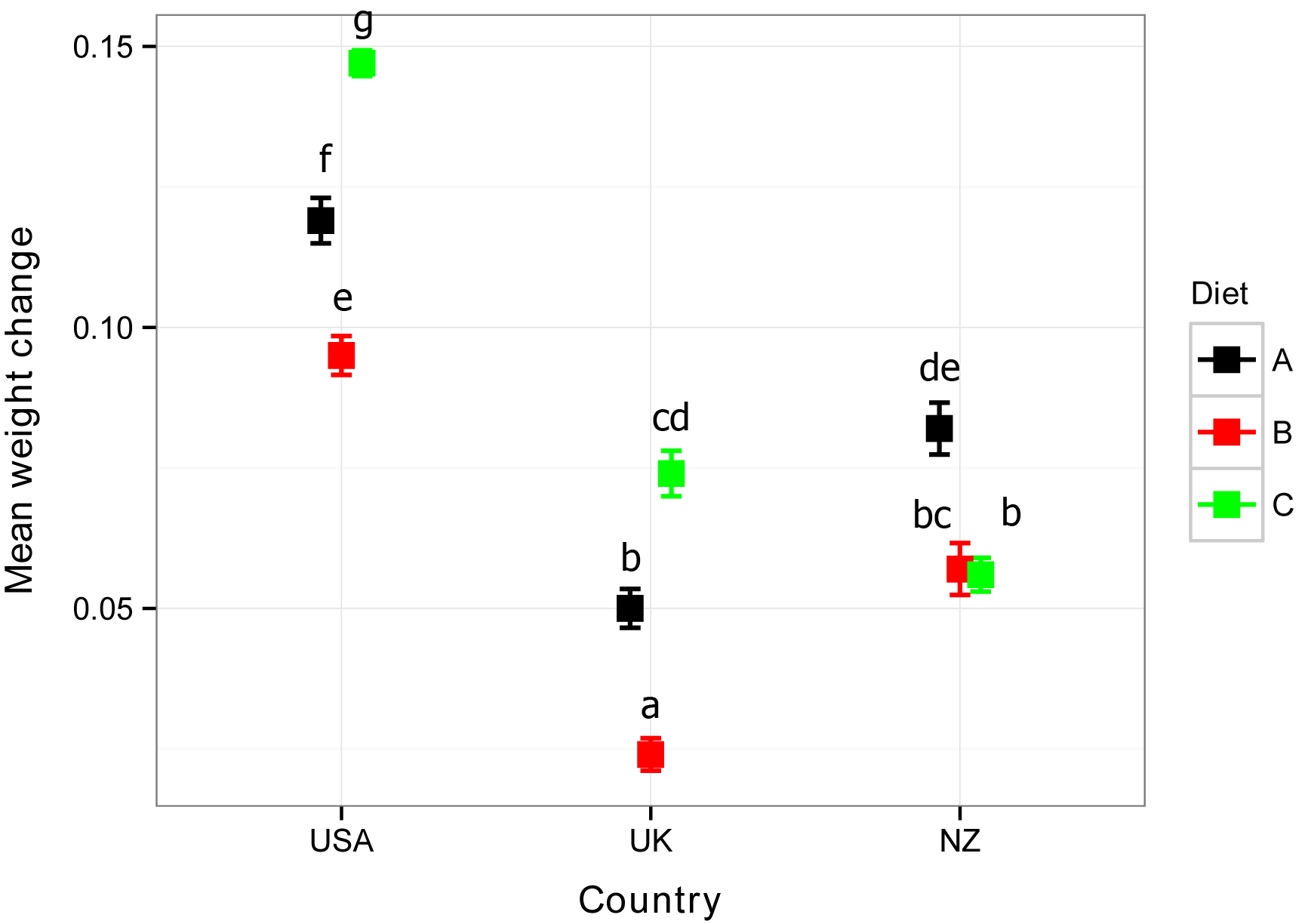## Summary and Analysis of Extension Program Evaluation in R

Salvatore S. Mangiafico

# Factorial ANOVA: Main Effects, Interaction Effects, and Interaction Plots

Two-way or multi-way data often come from experiments with a factorial design.  A factorial design has at least two factor variables for its independent variables, and multiple observation for every combination of these factors.

The weight gain example below shows factorial data.  In this example, there are three observations for each combination of Diet and Country.

With this kind of data, we are usually interested in testing the effect of each factor variable (main effects) and then the effect of their combination (interaction effect).

For two-way data, an interaction plot shows the mean or median value for the response variable for each combination of the independent variables.  This type of plot, especially if it includes error bars to indicate the variability of data within each group, gives us some understanding of the effect of the main factors and their interaction.

When main effects or interaction effects are statistically significant, post-hoc testing can be conducted to determine which groups differ significantly from other groups.  With a factorial experiment, there are a few guidelines for determining when to do post-hoc testing.  The following guidelines are presented for two-way data for simplicity.

•  When neither the main effects nor the interaction effect is statistically significant, no post-hoc mean-separation testing should be conducted.

•  When one or more of the main effects are statistically significant and the interaction effect is not, post-hoc mean-separation testing should be conducted on significant main effects only.
(This is shown in the first weight gain example below)

•  When the interaction effect is statistically significant, post-hoc mean-separation testing should be conducted on the interaction effect only.  This is the case even when the main effects are also statistically significant.
(This is shown in the second weight gain example below)

The final guideline above is not always followed.  There are times when people will present the mean-separation tests for significant main effects even when the interaction effect is significant.  In general, though, if there is a significant interaction, the mean-separation tests for interaction will better explain the results of the analysis, and the mean-separation tests for the main effects will be of less interest.

### Packages used in this chapter

The packages used in this chapter include:

•  psych

•  car

•  multcomp

•  emmeans

•  FSA

•  ggplot2

•  phia

The following commands will install these packages if they are not already installed:

if(!require(car)){install.packages("car")}
if(!require(psych)){install.packages("psych")}
if(!require(multcomp)){install.packages("multcomp")}
if(!require(emmeans)){install.packages("emmeans")}
if(!require(FSA)){install.packages("FSA")}
if(!require(ggplot2)){install.packages("ggplot2")}
if(!require(phia)){install.packages("phia")}

### Two-way ANOVA example with interaction effect

Imagine for this example an experiment in which people were put on one of three diets to encourage weight gain.  The amount of weight gained will be the dependent variable and will be considered an interval/ratio variable.  For independent variables, there are three different diets and the country in which subjects live.

The model will be fit with the lm function, whose syntax is similar to that of the clm function.

This kind of analysis makes certain assumptions about the distribution of the data, but for simplicity, this example will ignore the need to determine that the data meet these assumptions.

Diet    Country  Weight_change
A       USA      0.120
A       USA      0.125
A       USA      0.112
A       UK       0.052
A       UK       0.055
A       UK       0.044
B       USA      0.096
B       USA      0.100
B       USA      0.089
B       UK       0.025
B       UK       0.029
B       UK       0.019
C       USA      0.149
C       USA      0.150
C       USA      0.142
C       UK       0.077
C       UK       0.080
C       UK       0.066
")

### Order levels of the factor; otherwise R will alphabetize them

Data\$Country = factor(Data\$Country,
levels=unique(Data\$Country))

###  Check the data frame

library(psych)

str(Data)

summary(Data)

#### Simple interaction plot

The interaction.plot function in the native stats package creates a simple interaction plot for two-way data.  The options shown indicate which variables will used for the x-axis, trace variable, and response variable.  The fun=mean option indicates that the mean for each group will be plotted.  For the meaning of other options, see ?interaction.plot.

This style of interaction plot does not show the variability of each group mean, so it is difficult to use this style of plot to determine if there are significant differences among groups.

The plot shows that mean weight gain for each diet was lower for the UK compared with USA.  And that this difference was relatively constant for each diet, as is evidenced by the lines on the plot being parallel.  This suggests that there is no large or significant interaction effect.  That is, the difference among diets is consistent across countries.  And vice-versa, the difference in countries is consistent across diets.

A couple of other styles of interaction plot are shown at the end of this chapter.

interaction.plot(x.factor     = Data\$Country,
trace.factor = Data\$Diet,
response     = Data\$Weight_change,
fun = mean,
type="b",
col=c("black","red","green"),  ### Colors for levels of trace var.
pch=c(19, 17, 15),             ### Symbols for levels of trace var.
fixed=TRUE,                    ### Order by factor order in data
leg.bty = "o")#### Specify the linear model and conduct an analysis of variance

A linear model is specified with the lm function.  Weight_change is the dependent variable.  Country and Diet are the independent variables, and including Country:Diet in the formula adds the interaction term for Country and Diet to the model.

The ANOVA table indicates that the main effects are significant, but that the interaction effect is not.

model = lm(Weight_change ~ Country + Diet + Country:Diet,
data = Data)

library(car)

Anova(model,
type = "II")

Anova Table (Type II tests)

Sum Sq Df  F value    Pr(>F)
Country      0.022472  1 657.7171 7.523e-12 ***
Diet         0.007804  2 114.2049 1.547e-08 ***
Country:Diet 0.000012  2   0.1756    0.8411
Residuals    0.000410 12

#### Post-hoc testing with emmeans

Because the main effects were significant, we will want to perform post-hoc mean separation tests for each main effect factor variable.

For this, we will use the emmeans package.  The linear model under consideration is called model, created the lm function above.  The formula in the emmeans function indicates that comparisons should be conducted for the variable Country in the first call, and for the variable Diet in the second call.

library(emmeans)

marginal = emmeans(model,
~ Country)

pairs(marginal,

contrast  estimate      SE  df  t.ratio  p.value
USA - UK    0.0707 0.00276  12   25.646   <.0001

Results are averaged over the levels of: Diet

library(emmeans)

marginal = emmeans(model,
~ Diet)

pairs(marginal,

contrast estimate      SE df t.ratio p.value
A - B       0.025 0.00337 12   7.408  <.0001
A - C      -0.026 0.00337 12  -7.704  <.0001
B - C      -0.051 0.00337 12 -15.112  <.0001

Results are averaged over the levels of: Country
P value adjustment: tukey method for comparing a family of 3 estimates

#### Extended example with additional country

For the following example, the hypothetical data have been amended to include a third country, New Zealand.

Diet    Country  Weight_change
A       USA      0.120
A       USA      0.125
A       USA      0.112
A       UK       0.052
A       UK       0.055
A       UK       0.044
A       NZ       0.080
A       NZ       0.090
A       NZ       0.075
B       USA      0.096
B       USA      0.100
B       USA      0.089
B       UK       0.025
B       UK       0.029
B       UK       0.019
B       NZ       0.055
B       NZ       0.065
B       NZ       0.050
C       USA      0.149
C       USA      0.150
C       USA      0.142
C       UK       0.077

C       UK       0.080
C       UK       0.066
C       NZ       0.055
C       NZ       0.065
C       NZ       0.050
C       NZ       0.054
")

### Order levels of the factor; otherwise R will alphabetize them

Data\$Country = factor(Data\$Country,
levels=unique(Data\$Country))

###  Check the data frame

library(psych)

str(Data)

summary(Data)

#### Simple interaction plot

The plot suggests that the effect of diet is not consistent across all three countries.  While Diet C showed the greatest mean weight gain for USA and UK, for NZ it has a lower mean than Diet A.  This suggests there may be a meaningful or significant interaction effect, but we will need to do a statistical test to confirm this hypothesis.

interaction.plot(x.factor     = Data\$Country,
trace.factor = Data\$Diet,
response     = Data\$Weight_change,
fun = mean,
type="b",
col=c("black","red","green"),  ### Colors for levels of trace var.
pch=c(19, 17, 15),             ### Symbols for levels of trace var.
fixed=TRUE,                    ### Order by factor order in data
leg.bty = "o")#### Specify the linear model and conduct an analysis of variance

The ANOVA table indicates that the interaction effect is significant, as are both main effects.

model = lm(Weight_change ~ Country + Diet + Country:Diet,
data = Data)

library(car)

Anova(model,
type = "II")

Anova Table (Type II tests)

Sum Sq Df F value    Pr(>F)
Country      0.0256761  2 318.715 2.426e-15 ***
Diet         0.0051534  2  63.969 3.634e-09 ***
Country:Diet 0.0040162  4  24.926 2.477e-07 ***
Residuals    0.0007653 19

#### Post-hoc testing with emmeans

Because the interaction effect was significant, we would like to compare all group means from the interaction.  Even though the main effects were significant, the typical advice is to not conduct pairwise comparisons for main effects when their interaction is significant.  This is because focusing on the groups in the interaction better describe the results of the analysis.

We will use the emmeans package and ask for a compact letter display with the cld function.  First we create an object, named marginal, with the results of the call to emmeans.  Notice here that the formula indicates that comparisons should be conducted for the interaction of Country and Diet, indicated with Country:Diet.

Be sure to read the Estimated Marginal Means for Multiple Comparisons chapter for correct interpretation of estimated marginal means.  For lm model objects, the values for emmean, SE, LCL, and UCL values are meaningful.

library(emmeans)

marginal = emmeans(model,
~ Country:Diet)

pairs(marginal,

library(multcomp)

cld(marginal,
alpha=0.05,
Letters=letters,      ### Use lower-case letters for .group

Country Diet emmean      SE df lower.CL upper.CL .group
UK      B    0.0243 0.00366 19   0.0129   0.0358  a
UK      A    0.0503 0.00366 19   0.0389   0.0618   b
NZ      C    0.0560 0.00317 19   0.0461   0.0659   b
NZ      B    0.0567 0.00366 19   0.0452   0.0681   bc
UK      C    0.0743 0.00366 19   0.0629   0.0858    cd
NZ      A    0.0817 0.00366 19   0.0702   0.0931     de
USA     B    0.0950 0.00366 19   0.0836   0.1064      e
USA     A    0.1190 0.00366 19   0.1076   0.1304       f
USA     C    0.1470 0.00366 19   0.1356   0.1584        g

Confidence level used: 0.95
Conf-level adjustment: sidak method for 9 estimates
P value adjustment: tukey method for comparing a family of 9 estimates
significance level used: alpha = 0.05

### Groups sharing a letter are not significantly different
###   at the alpha = 0.05 level.

#### Interaction plot with error bars using ggplot2

The interaction plots created with the interaction.plot function above are handy to investigate trends in the data, but have the disadvantage of not showing the variability in data within each group.

The package ggplot2 can be used to create attractive interaction plots with error bars.  Here, we will use standard error of each mean for the error bars.

### Create a data frame called Sum with means and standard deviations

library(FSA)

Sum = Summarize(Weight_change ~ Country + Diet,
data=Data,
digits=3)

### Add standard error of the mean to the Sum data frame

Sum\$se = Sum\$sd / sqrt(Sum\$n)

Sum\$se = signif(Sum\$se, digits=3)

Sum

Country Diet n nvalid  mean    sd   min    Q1 median    Q3   max percZero      se
1     USA    A 3      3 0.119 0.007 0.112 0.116  0.120 0.122 0.125        0 0.00404
2      UK    A 3      3 0.050 0.006 0.044 0.048  0.052 0.054 0.055        0 0.00346
3      NZ    A 3      3 0.082 0.008 0.075 0.078  0.080 0.085 0.090        0 0.00462
4     USA    B 3      3 0.095 0.006 0.089 0.092  0.096 0.098 0.100        0 0.00346
5      UK    B 3      3 0.024 0.005 0.019 0.022  0.025 0.027 0.029        0 0.00289
6      NZ    B 3      3 0.057 0.008 0.050 0.052  0.055 0.060 0.065        0 0.00462
7     USA    C 3      3 0.147 0.004 0.142 0.146  0.149 0.150 0.150        0 0.00231
8      UK    C 3      3 0.074 0.007 0.066 0.072  0.077 0.078 0.080        0 0.00404
9      NZ    C 4      4 0.056 0.006 0.050 0.053  0.054 0.058 0.065        0 0.00300

### Order levels of the factor; otherwise R will alphabetize them

Sum\$Country = factor(Sum\$Country,
levels=unique(Sum\$Country))

### Produce interaction plot

library(ggplot2)

pd = position_dodge(.2)

ggplot(Sum, aes(x = Country,
y = mean,
color = Diet)) +
geom_errorbar(aes(ymin = mean - se,
ymax = mean + se),
width=.2, size=0.7, position=pd) +
geom_point(shape=15, size=4, position=pd) +
theme_bw() +
theme(axis.title = element_text(face = "bold")) +

scale_colour_manual(values= c("black","red","green")) +
ylab("Mean weight change")##### Interaction plot with mean separation letters manually added

It is common to add mean separation letters from post-hoc analyses to interaction plots.  One option is to add letters manually in either image manipulation software like Photoshop or GIMP, or in a word processor or other software that can handle graphic manipulation.

Letters can also be added to the plot by ggplot2 with the annotate or geom_text options.  See “Optional:  Interaction plot of estimated marginal means with mean separation letters” in the Estimated Marginal Means for Multiple Comparisons chapter for examples.

In some cases it is desirable for means to be lettered so that the greatest mean is indicated with a.  However, emmeans by default labels the least mean with a.  The order of letters can be reversed manually, or the reversed=TRUE option can be used.Plot of mean weight change for three diets in three countries.  Means sharing a letter are not significantly different according to pairwise comparisons of estimated marginal means with Tukey adjustment for multiple comparisons.

#### Interaction plot with the phia package

The phia package can be used to create interaction plots quickly.  By default the error bars indicate standard error of the means.  For additional options, see ?interactionMeans.

Note that model is the linear model specified above.

library(phia)

IM = interactionMeans(model)

IM

Country Diet adjusted mean  std. error
1     USA    A    0.11900000 0.003664274
2      UK    A    0.05033333 0.003664274
3      NZ    A    0.08166667 0.003664274
4     USA    B    0.09500000 0.003664274
5      UK    B    0.02433333 0.003664274
6      NZ    B    0.05666667 0.003664274
7     USA    C    0.14700000 0.003664274
8      UK    C    0.07433333 0.003664274
9      NZ    C    0.05600000 0.003173354

### Produce interaction plot

plot(IM)

### Return the graphics device to its default 1-plot-per-window state

par(mfrow=c(1,1))